# Lesson 4: Fading an LED

In the previous lesson, we learned how to turn on and off an LED using digitalWrite—which worked by alternatively setting Pin 3 to 5V (HIGH) and 0V (LOW). In this lesson, we’ll learn how to programmatically control the output voltage at finer gradations using analogWrite. More specifically, we will gradually fade an LED on and off like the animation below illustrates.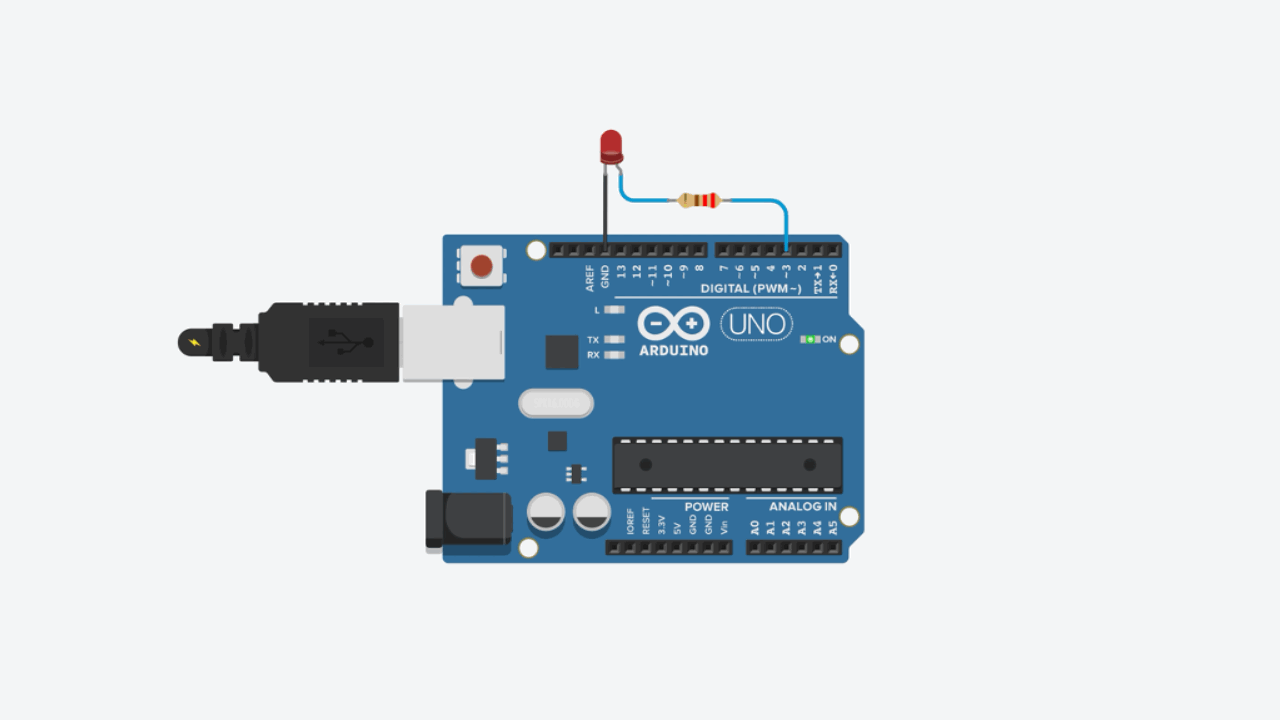This illustrative animation doesn’t show current (the yellow circles) only due to my limited animation skills. But hopefully you can visualize (in your mind) how the LED varies in brightness with current just the same. :)

## Materials

You will use the same materials as before, including the Arduino IDE and a USB cable to upload your program from your computer to your Arduino.

Arduino LED Resistor
Arduino Uno, Leonardo, or similar Red LED 220Ω Resistor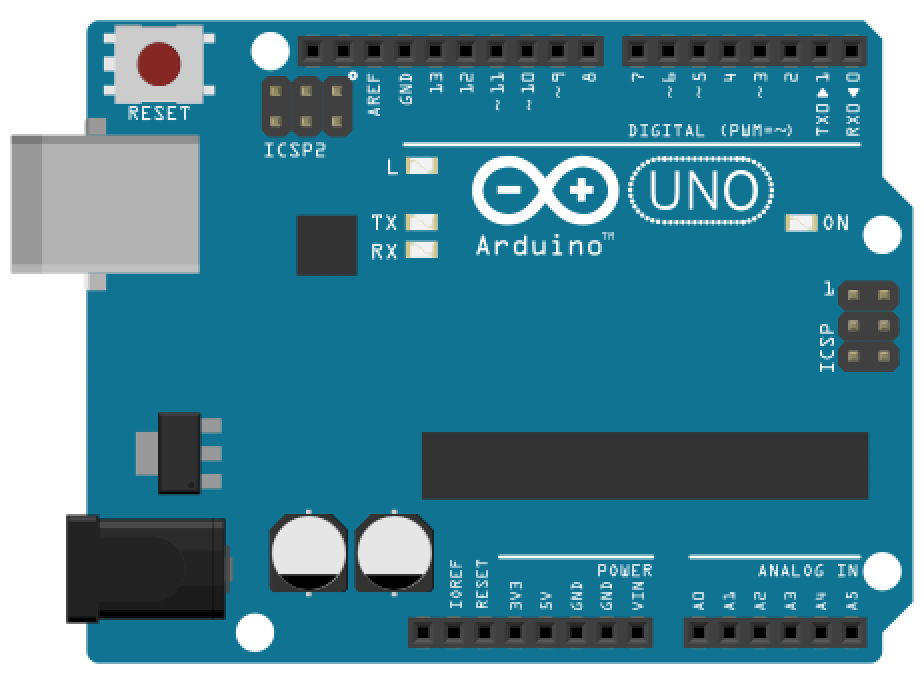Arduino Uno, Leonardo, or similar Red LED 220Ω Resistor

## Making the circuit

As noted in our previous lesson, the Arduino Uno has 14 digital I/O pins: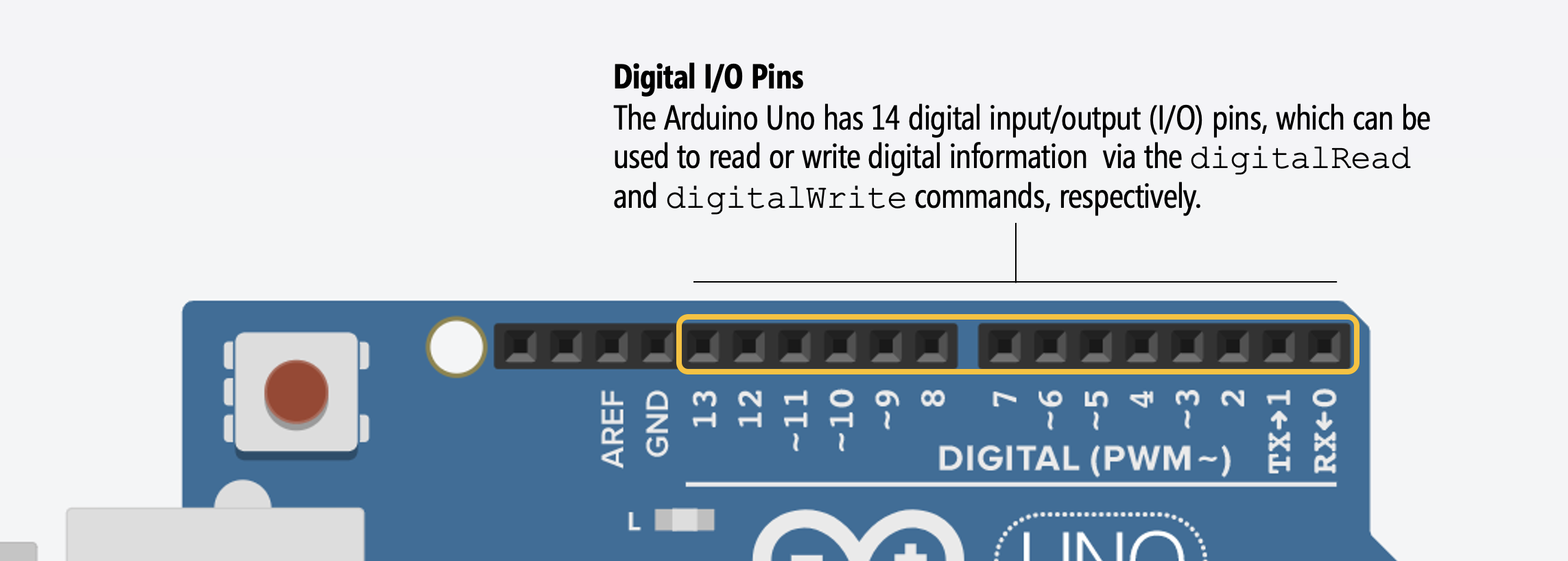However, 6 of the 14 I/O pins can also be used for “analog” output—voltage output that is not just HIGH (5V) or LOW (0V) but between these two extremes. These analog output pins are indicated by the tilde (~) printed next to the pin on the Arduino (silkscreened directly on the Arduino’s PCB).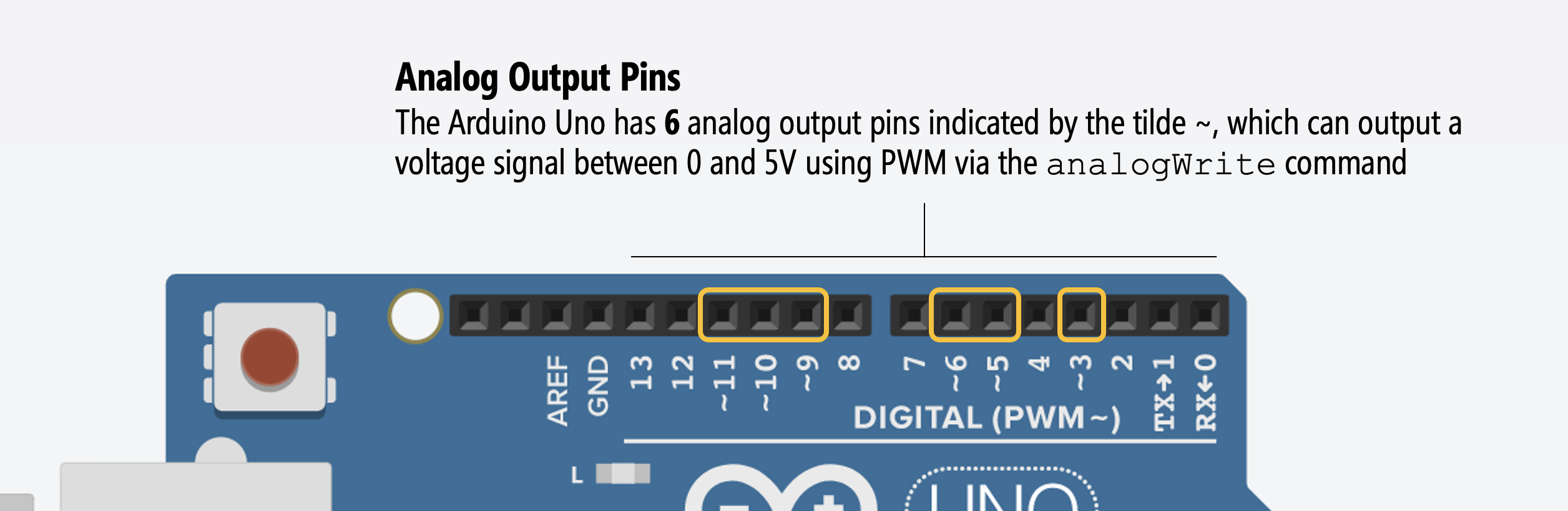So, for this lesson, we don’t have to change our circuit at all! You can keep the same circuit as the LED Blink lesson. Indeed, this is the reason why we selected Pin 3 in the first place.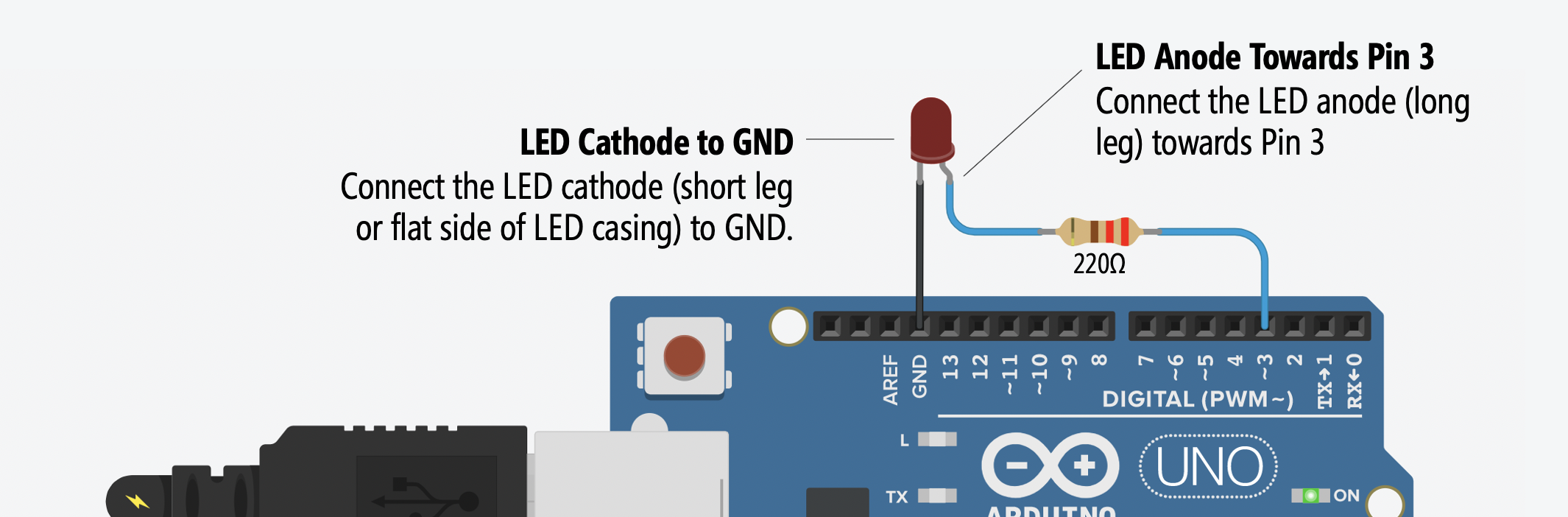### A common confusion: analog I/O pins are different!

A common confusion amongst beginners is mixing up the analog output pins and the analog input pins. For digital I/O, the input and output pins are the same and configurable to INPUT or OUTPUT using the pinMode command, the analog I/O pins are different! See the figure below: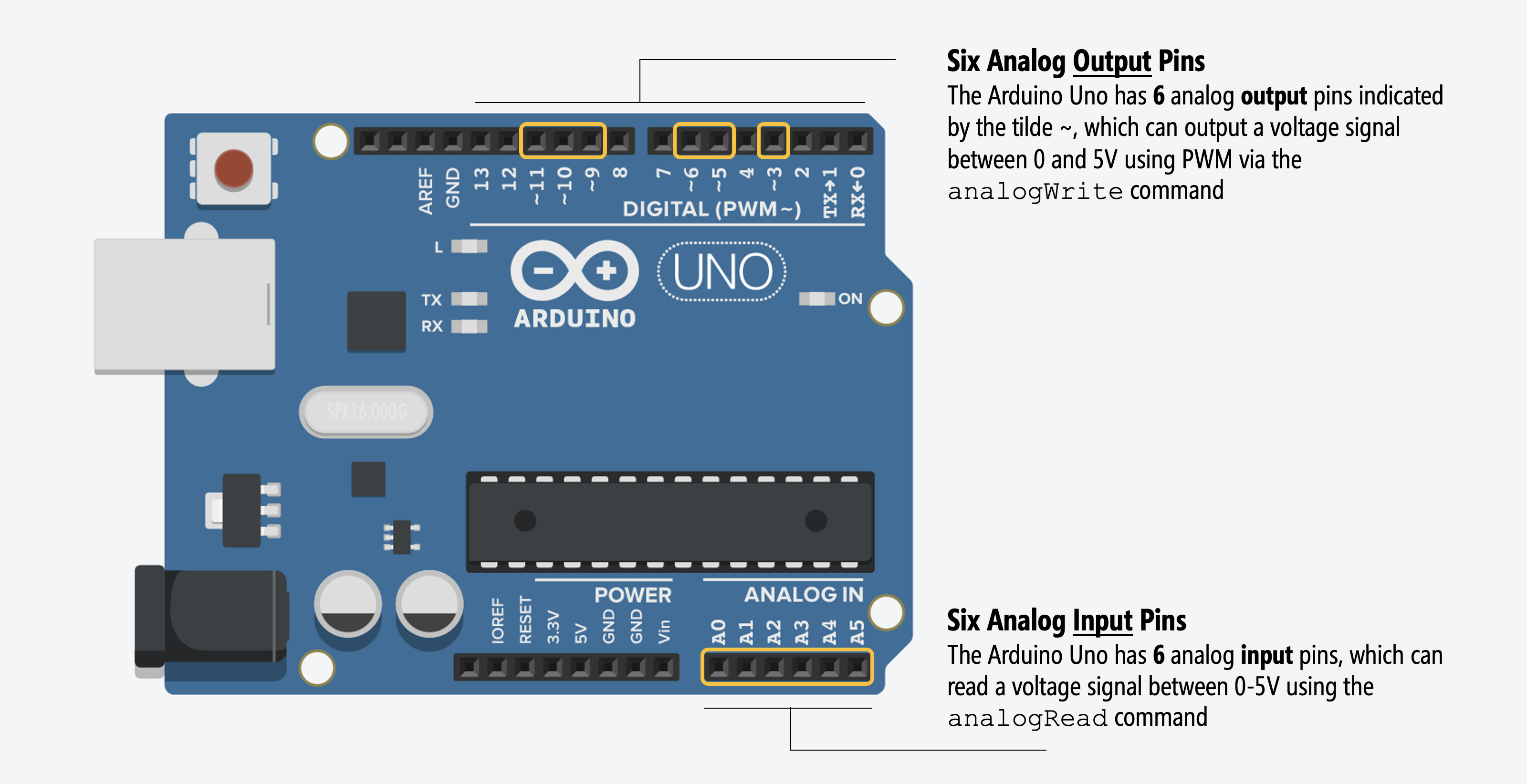We’ll learn about analog output in this lesson (using analogWrite). In a future lesson, we will learn about analog input (using analogRead)

## Using analogWrite

To gradually fade an LED, we are going to use the analogWrite(int pin, int value) function, which takes in a pin as the first parameter and an 8-bit value between 0-255 as the second.

### Pulse-width modulation (PWM)

Despite its name, the Arduino Uno, Leonardo, Nano, Mega, and many other Arduino boards do not actually provide true analog output via a digital-to-analog converter (DAC). Instead, they use a method called Pulse-Width Modulation (PWM) to emulate analog output. For most purposes—like changing the brightness of an LED or controlling the speed of a motor—this won’t matter; however, if you want to output a high-frequency sinusoidal waveform—a true analog output signal—like playing music, then you’ll need to either find an Arduino microcontroller with a built-in DAC like the Due (see this SimpleAudioPlayer tutorial) or connect your Uno to an external DAC board like this SparkFun MP3 Player Shield.

So, what does the analogWrite function do, exactly? The 8-bit value (0-255) directly controls how long a 5V value is applied to the output pin during one “analog write” period. So, analogWrite(<pin>, 127) would output a 5V value for half the period (because 127/255 = ~50%) and analogWrite(<pin>, 191) would output a 5V for 75% of the period (because 191/255 = ~75%). This fraction of the time the signal is HIGH is called the duty cycle.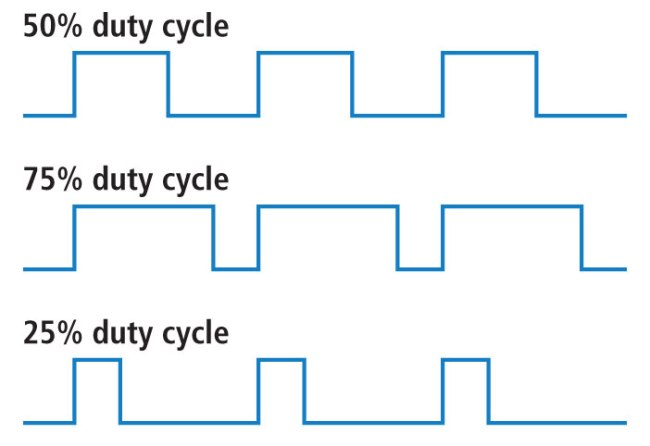Pulse-width modulation duty cycle graph from Sparkfun’s PWM Tutorial

Why does the Arduino Uno only have six PWM outputs? Because the ATmega328 microcontroller has three hardware timers, which control the six PWM outputs.

Could I manually implement PWM on any pin simply by rapidly turning the pin on and off at a desired frequency and duty cycle? Yes, however, the PWM waveform could be jittery (unless you disable interrupts). See: SecretsOfArduinoPWM and example code that manually implements a PWM loop.

## Writing the code

OK, so let’s write some code!

### Step 1: Start a new sketch in the Arduino IDE

Start a new sketch in the Arduino IDE: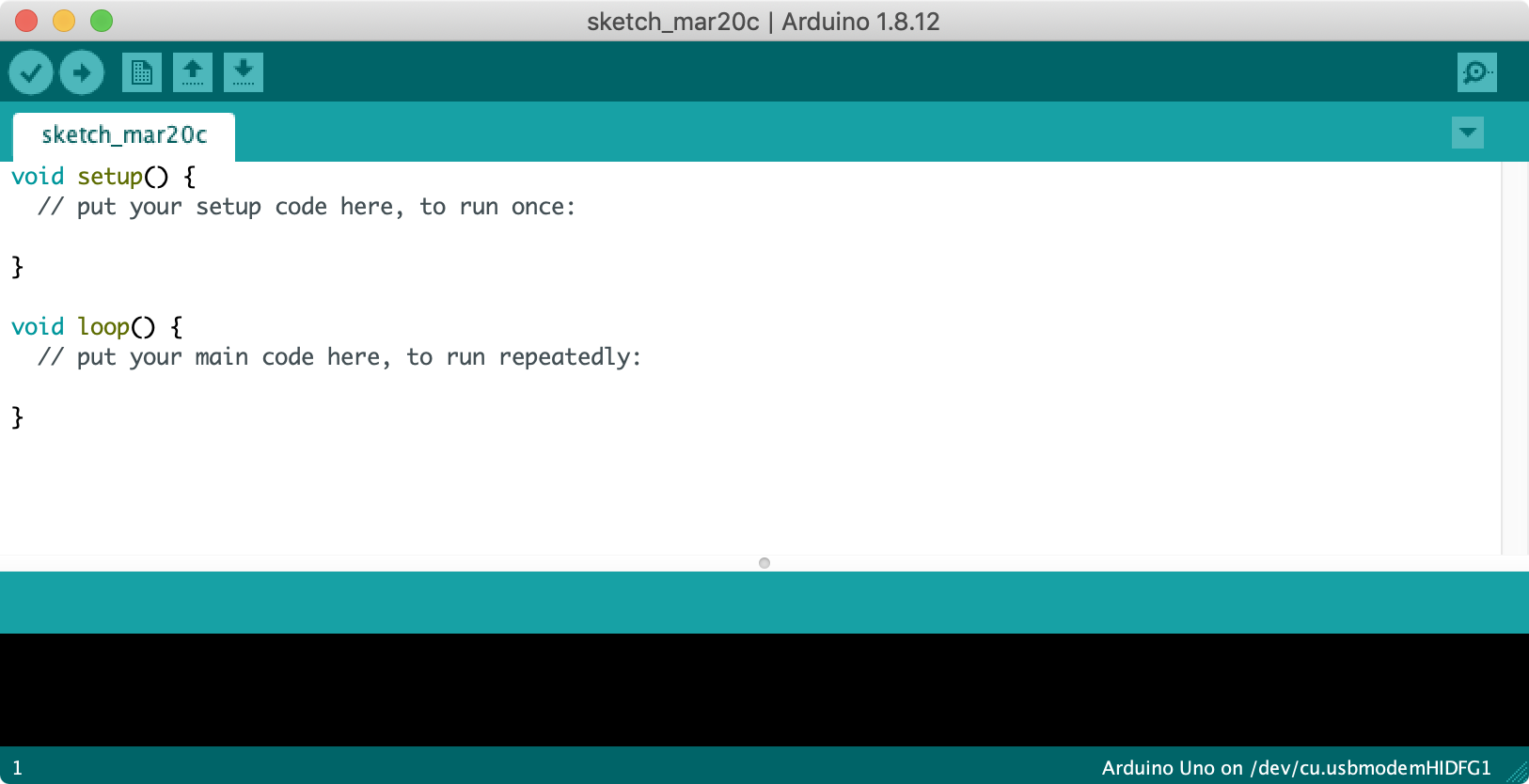### Step 2: Write initialization code

Our initialization code is the same as for LED blink except for the addition of const int MAX_ANALOG_OUT = 255; and a constant for the delay amount of 5 milliseconds (const int DELAY_MS = 5;).

### Step 3: Write fade loop

Now, write code that outputs steadily increasing values for analogWrite (to fade on) followed by steadily decreasing values (to fade off).

The full code is embedded below:

This source code is on GitHub.

### Step 4: Compile, upload, and run!

Now, compile, upload, and run the code. After upload completes, the LED should immediately begin fading on then off. See video below.

## Visualizing the voltage output

What’s actually happening on Pin 3 when we write out different values to analogWrite? Well, remember, basic Arduino boards like the Uno and Leonardo do not have the ability to write out intermediate voltages (they lack digital-to-analog converters or DACs). So, instead, they “fake it” by using pulse-width modulation (PWM) and modulating the fraction of time that a 5V output waveform is HIGH vs. LOW. This is called the duty cycle.

### Visualizing the PWM waveform

To let you see how the PWM waveform changes with different analogWrite values, we wrote a simple program that takes in an analog input (from a potentiometer, in this case) and uses it to set an analogWrite value to Pin 3. Other than the potentiometer, our circuit did not change (we still have an LED with a current-limiting resistor on Pin 3).

Let’s take a look:

To create this program, we had to use both analogRead and analogWrite. By the end of this lesson, you should have a strong understanding of analogWrite and PWM. But we won’t learn more about analogRead until we get to the Introduction to Input microcontroller lessons.

### Visualizing the effective voltage output

In addition to visualzing the actual voltage output from analogWrite (the PWM waveform), we can also visualize the (effective) voltage output. For this, we can use Arduino’s Serial Plotter. To access this, open Tools -> Serial Plotter. The plotter will try to visualize any comma separated values you output via Serial.print.

In the video below, we see a simulation of our fade code + circuit running in Tinkercad. On the right side, in the Serial Monitor window, we are printing and graphing out the real-time effective voltages output on Pin 3.

## Calculating the current through our LED

Of course, it’s the current through the LED that determines brightness. But how can we calculate (effective) current with PWM?

Again, given Ohm’s Law ($$I = \frac{V}{R}$$), we can determine the current through our circuit at various Pin 3 outputs. Recall that current does not pass through an LED until its forward voltage $$V_f$$ condition is met. With a red LED, a common forward voltage is $$V_f=2V$$. With analogWrite, the GPIO pin is still driven HIGH (5V) but only for a fraction of the time due to PWM (this fraction is called the duty cycle). So, the $$V_f$$ requirement is still met and our eyes perceive the LED on but it’s actually flashing on/off imperceptibly quickly!

To calculate the current through our LED circuit with PWM, let’s let $$DF$$ equal the duty cycle fraction, the fraction of a waveform period that is HIGH. Then, we can use the following equation: $$I = \frac{V_s - V_f}{R} * DF$$ to calculate current. So, for example, if we analogWrite a 51 then our $$DF=\frac{51}{255}=0.2$$. With a 220Ω, our current would be: $$I=\frac{5V - 2V}{220Ω}*0.2=2.7mA$$.

See the table below for example 8-bit output values for analogWrite on Pin 3 and the effective current.

Resistor Pin 3 Voltage Pin 3 Value PWM Duty Cycle Resulting Current
220Ω 5V 0 $$\frac{0}{255}=0.0$$ $$I = \frac{5V-2V}{220Ω} * 0.0=0.0mA$$
220Ω 5V 45 $$\frac{45}{255}=0.176$$ $$I = \frac{5V-2V}{220Ω} * 0.176=2.4mA$$
220Ω 5V 103 $$\frac{103}{255}=0.404$$ $$I = \frac{5V-2V}{220Ω} * 0.404=5.5mA$$
220Ω 5V 128 $$\frac{128}{255}=0.502$$ $$I = \frac{5V-2V}{220Ω} * 0.780=6.8mA$$
220Ω 5V 199 $$\frac{199}{255}=0.780$$ $$I = \frac{5V-2V}{220Ω} * 0.780=10.5mA$$
220Ω 5V 255 $$\frac{255}{255}=1.0$$ $$I = \frac{5V-2V}{220Ω} * 1.0=13.4mA$$

## Improved fading approach: removing for loop

Remember in the LED blink lesson where we mentioned wanting to avoid long for loops and long delays in our code. Why? Because while we are in a delay, we can’t do anything else: we can’t read or respond to other input (side note: we could use interrupts but let’s defer that point for now). See “What does delay() actually do?” in our Inside Arduino guide.

So, let’s rewrite the fade example but without for loops and, instead, rely on the fact that loop() is already a loop :). While the code below is different, the resulting LED fade behavior is the same (so you won’t notice a difference if you try them both out).

NOTE:

I have a habit of prefixing my global variables by _ but this is just my own convention and helps me easily discern between local variables and global variables.

You can find this code in GitHub.

## Improved fading approach 2: eliminating delays

Can you improve the above code even more? How about by eliminating the delay() all together but still allowing for a set interval to “pause” on each LED fade value?

Try writing a solution yourself then look at ours. Are they the same or different? How?

## Next Lesson

In the next lesson, we will learn about the difference between current sources and current sinks to help reinforce our understanding of how microcontrollers can control output.

This website was developed by Professor Jon E. Froehlich and the Makeability Lab using Just the Docs. If you found the website useful or use it in your teaching, we'd love to hear from you: jonf@cs.uw.edu. This website and all code is open source (website GitHub, Arduino GitHub, p5js GitHub). You can find the MakeabilityLab_Arduino_Library here. Found an error? File a GitHub Issue.